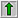You are here
Home > Medical Calculator > Ireton-Jones Energy requirements -critical care

## Determination of the Resting Energy Expenditure in Hospitalized / Critically ill Patients## Background

Equations are listed below.   The final selection of equations was based on  information found within the ADA evidence library and the following reference:

Walker RN, Heuberger RA. Predictive Equations for Energy Needs for the Critically Ill. Respir Care 2009;54(4):509:521.

Direct quotes:    "Understanding an equations reference population and using the equation with similar patients are essential for the equation to perform similarly. Prediction accuracy among equations is rarely within 10% of the measured energy expenditure; however, in the absence of indirect calorimetry, a prediction equation is the best alternative."       "However, if an equation must be used, the 1998 and 2003 Penn State equations, the 1992 Ireton-Jones equation, and the Swinamer equation are worthy of consideration."

Important note:  during the next update, additional equations may be added. This initial version will only calculate results for the Ireton-Jones equations.

Age     Gender:

Weight

## Select all that apply## Dieting - BMR - RMR Calculators:

Several of these calculators may be particularly useful for dieters.  Just about every single MAJOR calorie/ energy equation that has been released over the last 90 years is included below.   Each calculator has a customized printout option for easy analysis.  Recommendation: Try each calculator - print out the results -  then compare!

Estimated 'Calorie' Calculators:

Harris-Benedict Equation:  Estimation of total calories needed. MOST widely used equation for calculating basal metabolic rate and total calories.
Revised Harris-Benedict Equation:The original Harris Benedict equation was revised in 1984.  This updated equation can be used to calculate the basal metabolic rate and total calories.
RESTING Metabolic Rate (RMR): Resting Metabolic Rate Calc - This equation can be used to calculate the RESTING metabolic rate and total calories. Mifflin-St Jeor equation.
Schofield equation (BMR) : This equation was part of the previous government guidelines to formulate RDA's and can be used to calculate the basal metabolic rate and total calories needed.
Institute of Medicine Equation- LATEST EQUATION: IOM Equation-Estimated Energy Requirement (EER) Estimation of total calories needed. This equation is behind the 2005 Dietary Guidelines for Americans and the new food pyramid, MyPyramid.

Check out the new BMR multi-calc.

## Ireton-Jones Energy Equations IJEE:

Ventilator-dependent:
IJEE(v) = 1784 - 11(A)+ 5(W)+244 (G) + 239(T) + 804(B)
2002 version (revised 1997 equation).

IJEE(v) = 1925 - 10(A) + 5(W) +281 (G) +292 (T) +851 (B)
1992 version

Spontaneously Breathing:
IJEE(s) = 629 - 11 (A) + 25 (W) - 609 (O)

IJEE = kcal/day; A = age (yrs); W = actual wt(kg); G = gender(male=1, female=0); T = trauma, B = burn, O=obesity( if present=1, absent=0)

## Other equations to consider

Harris Benedict Equations must be used with a custom stress factor:
BMR calculation for men (metric)

BMR = 66.47 + ( 13.75 x weight in kg ) + ( 5.003 x height in cm ) - ( 6.755 x age in years )

BMR calculation for women (metric)
BMR = 655.1 + ( 9.563 x weight in kg ) + ( 1.850 x height in cm ) - ( 4.676 x age in years )

Reference: Harris JA, Benedict FG. A biometric study of human basal metabolism. Proc Natl Acad Sci USA 1918;4(12):370-3.

Penn State Equations:
Penn State 1998 equation
(1.1 x value from Harris-Benedict equation - use ajbw if obese) + (140 x Tmax)+ (32 x VE) - 5340

Penn State 2003 equation
(0.85 x value from Harris-Benedict equation) + (175 x Tmax) + (33 x VE) - 6,433

Reference:
Frankenfield D, Smith S, Cooney RN. Validation of 2 approaches to predicting resting metabolic rate in critically ill patients. JPEN J Parenter Enteral Nutr 2004;28(4):259-264.

Swinamer 1990 equation:
(945 x body surface area) - (6.4 x age) + (108 x temp in celsius) + (24.2 x respiratory rate in breaths/min) + (817 x VT) - 4349

Reference:
Swinamer DL, Grace MG, Hamilton SM, Jones RL, Roberts P, King G. Predictive equation for assessing energy expenditure in mechanically ventilated critically ill patients. Crit Care Med 1990;18(6):657-661.

## ReferenceIreton-Jones C, Jones JD. Improved equations for predicting energy expenditure in patients: The Ireton-Jones equations. Nutr Clin Pract. 2002;17:29-31.

Ireton-Jones CA, Turner WW, Liepa GU, Baxter CR. Equations for the estimation of energy expenditures in patients with burns with special reference to ventilatory status. J Burn Care Rehabil 1992; 13(3):330-333.  https://www.carolireton-jones.com/

﻿